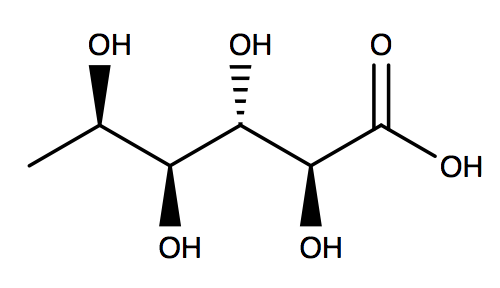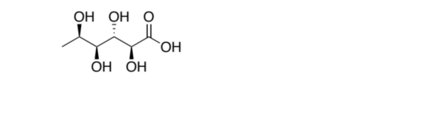# Problem: Convert the following bond line formula into a Fischer projection.

###### FREE Expert Solution
95% (114 ratings)
###### FREE Expert Solution

We’re being asked to convert the given bondline structure into a Fischer projection.Recall that Fischer projections are a way of drawing stereoisomers, usually for sugars and carbohydrates. In a Fischer projection:

• Horizontal lines: are above the plane or in a wedge line

• Vertical lines: are below the plane or in a dashed line

• Intersections: are chiral centers

95% (114 ratings)###### Problem Details

Convert the following bond line formula into a Fischer projection.